### UPPER Function Examples – Excel & Google Sheets

This tutorial demonstrates how to use the Excel UPPER Function in Excel to convert text to upper case.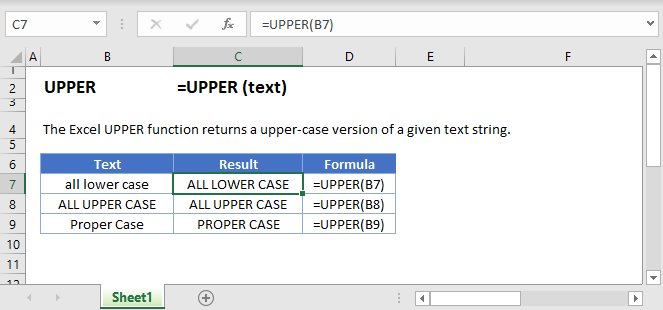## UPPER Function Overview

The UPPER Function Converts entire text string to upper case.

To use the UPPER Excel Worksheet Function, select a cell and type: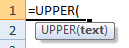(Notice how the formula inputs appear)

### UPPER function Syntax and inputs:

`=UPPER(text)`

text – A string of text.

AutoMacro - VBA Code Generator

## What Is the UPPER Function?

The Excel UPPER Function takes a string as an input, and then returns the same string in upper case.

## How to Use the UPPER Function

Use the Excel UPPER function like this:

`=UPPER(“usa”)`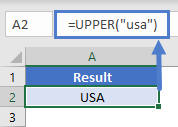This formula would return the following:

USA

See below for a few more examples:

`=UPPER(A2)`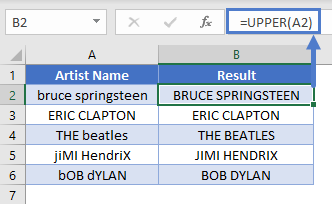## How UPPER Handles Numbers and Special Characters

The Excel UPPER Function has no effect on numbers, punctuation marks, or other special characters.

### How UPPER Handles Accented Letters

Generally speaking, if you use UPPER on a text string that contains lower case, accented letters, Excel will return an accented capital if one exists. However, UPPER is somewhat intelligent in how it goes about this.

See the following examples: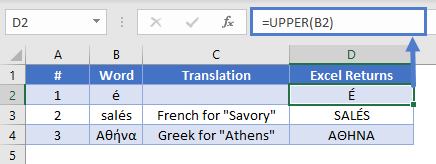In examples #1 and #2, we see that Excel capitalizes the accented letter whether it is alone or part of a word. This is worth keeping in mind, as in some language like French and Portuguese, upper case letters are sometimes not accented, depending on the style guide being followed.

In Greek, where words that appear entirely in upper case should not be accented, UPPER does not accent the capitalized letters, as you can see in examples #3, where ή is correctly transformed to H, not its accented version Ή.

### Using UPPER to count characters within a cell regardless of case

Say you need to count the number of times a particular character appears in a string, but you don’t care whether it’s upper or lower case. To do this, you can combine UPPER with LEN and SUBSTITUTE.

Look at these examples:

`=LEN(B2)-LEN(SUBSTITUTE(B2,C2,""))`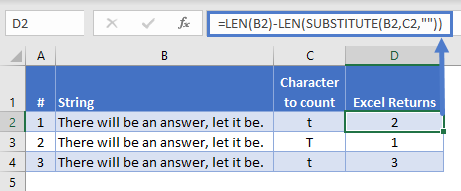In example #1, we use LEN to count the number of characters in B2 – which happens to be 35 characters.

Then, we use SUBSTITUTE to go through B2, look for any character that matches whatever’s in C2 – in this case, a lower-case t – and replace them with an empty string. In effect, we remove them. Then we pass the result to LEN, which happens to be 33.

The formula is subtracting the second calculation from the first: 35 – 33 = 2. This means a lower-case t appears in the B2 twice.

In example #2, we’ve done the same thing, but we’re looking for an upper-case T – and we find one.

But if we want to find upper OR lower case Ts, we need to use UPPER. You can see this in example #3 – within the substitute part of the formula, we convert both B4 and C4 to upper case – so this time, we find three matches.

## UPPER in Google Sheets

The UPPER Function works exactly the same in Google Sheets as in Excel: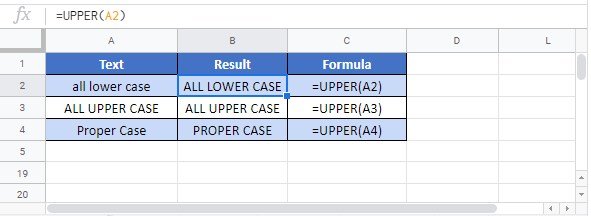## Additional Notes

The UPPER Function and LOWER Function are useful when working with case-sensitive Excel Functions such as Find Function and SUBSTITUTE Function. <>

Return to the List of all Functions in Excel

## Excel Practice Worksheet

Practice Excel functions and formulas with our 100% free practice worksheets!

• Automatically Graded Exercises
• Learn Excel, Inside Excel!